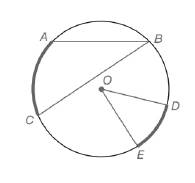Chapter 6.1, Problem 1EElementary Geometry For College St...

7th Edition
Alexander + 2 others
ISBN: 9781337614085

Solutions

Chapter
SectionElementary Geometry For College St...

7th Edition
Alexander + 2 others
ISBN: 9781337614085
Textbook Problem

For Exercises 1 to 8, use the figure provided.Exercises 1-8If m A C ⌢ = 58 ∘ ,  find m ∠ B .

To determine

To calculate:

The mB, by using the provided figure.

Explanation

Given:

The provided figure is given below:

Theorem used:

The measure of an inscribed angle of a circle is one-half the measure of its intercepted arc.

Calculation:

Given, mAC=58o.

Since, the measure of an inscribed angle of a circle is one-half the measure of its intercepted arc.

mB=12mAC

Still sussing out bartleby?

Check out a sample textbook solution.

See a sample solution

The Solution to Your Study Problems

Bartleby provides explanations to thousands of textbook problems written by our experts, many with advanced degrees!

Get Started

Explain what happens during each of the two stages of the two-factor ANOVA.

Essentials of Statistics for The Behavioral Sciences (MindTap Course List)

Simplify x3+x2. 5x6 5x12 2x5 x5

Trigonometry (MindTap Course List)

True or False: These lines are skew:

Study Guide for Stewart's Multivariable Calculus, 8th

For what values of p does the series converge?

Study Guide for Stewart's Single Variable Calculus: Early Transcendentals, 8th

Define the validity of measurement and explain why and how it is measured.

Research Methods for the Behavioral Sciences (MindTap Course List)

Distinguish between a hypothesis and a prediction.

Research Methods for the Behavioral Sciences (MindTap Course List)